Next: Solution Example II Up: mechanics Previous: mechanics

# Solution Example I

We first consider the vector transformation from the coordinate system K to the co-rotational system K'. The pointer vector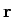is represented by the linear combination of the unit vector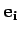(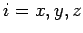) in K: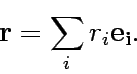(1)

Equally one can write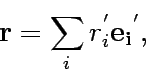(2)

in the co-rational system K'. The time differential of the unit vectors in K' is given by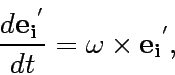(3)

where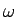is the angler velocity vector. When the differential operator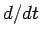is applied in equation (2), the relation (3) above gives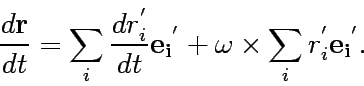(4)

Here the first term in the right hand side above is the velocity seen by the observer in the co-rational system K'. Consequently the equation (4) can read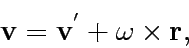(5)

where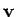is the velocity vector.

The acceleration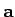in K is then obtained by the time differential of the equation (4) as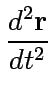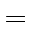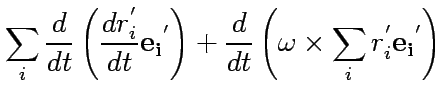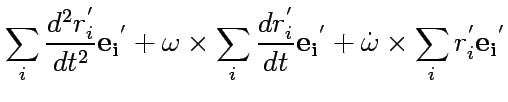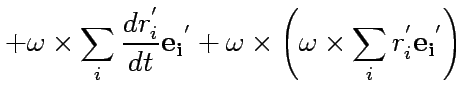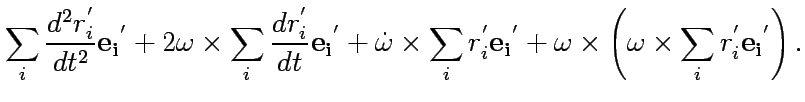(6)

Here we used the relation (3). The first term in the right hand side in the equation above represents the accelerator for the observer in K'. We, therefore, finally get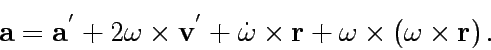(7)

The equation described above and the Newtonian equation of the motion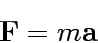(8)

then lead to the following final equation of the motion for the observer in k';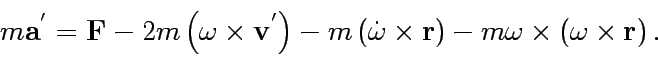(9)

The terms in the right hand side imply the external force, the coliori Force, the apparent force due to the angular (de-)acceleration, and the centrifugal force, respectively.Next: Solution Example II Up: mechanics Previous: mechanics
Shigeru Yoshida
2003-01-15